Definitions of Square Dance Calls and Concepts

Index -->  Plus  |  A1  |  A2  |  C1  |  C2  |  C3A  |  C3B  |  C4  |  NOL  |
Definitions (Text Only) -->  Plus  |  A1  |  A2  |  C1  |  C2  |  C3A  |  C3B  |  C4  |  NOL  |
 Find call:

Completed Double Pass Thru \$B\$+\$i(B.

Tandem Partner Trade; Extend \$B\$r(B n \$B2s\$7\$^\$9(B.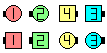\$B\$B;O\$a\$N(B formation: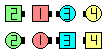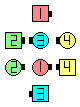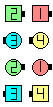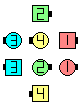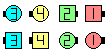Track 0\$B\$N8e(B Track 1\$B\$N8e(B Track 2\$B\$N8e(B Track 3\$B\$N8e(B Track 4\$B\$N8e(B

\$BCm
• Track n \$B\$O(B Completed Double Pass Thru \$B\$+\$i\$N\$_9T\$(\$^\$9(B. \$BJ?9T\$J(B Wave \$B\$d(B Mini-Wave \$B\$N(B Columns \$B\$N\$h\$&\$J(B formation \$B\$+\$i(B Track n \$B\$r%3!<%k\$9\$k\$3\$H\$O(B, \$BE,@Z\$G\$"\$j\$^\$;\$s(B.
• Track \$B\$O(B Tagging call \$B\$H\$7\$F;H\$o\$l\$^\$9(B.
• Track 2 [Plus] (Dick Bayer 1976): From completed Double Pass Thru. Tandem Partner Trade; Extend twice. Ends in Parallel R-H Waves.Choreography for Track nComments? Questions? Suggestions?